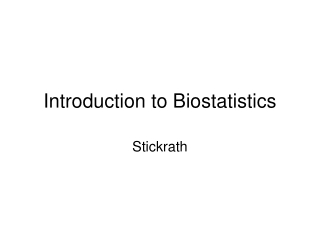DownloadDownload PresentationIntroduction to Biostatistics

# Introduction to Biostatistics

Download Presentation## Introduction to Biostatistics

- - - - - - - - - - - - - - - - - - - - - - - - - - - E N D - - - - - - - - - - - - - - - - - - - - - - - - - - -
##### Presentation Transcript

1. Introduction to Biostatistics Stickrath

2. Why do I need to understand statistics? • A group wanted to figure out which kinds of schools were good, so they compared all schools and looked at those for which a very large percentage of the students scored high on national exams or got into top universities. They found that SMALL schools (those with few students) were the ones that had the highest percentages of students excelling.

3. Raw data

4. Processed Data

5. Big Ideas • Sample Size • Extraneous Variables • Standard Deviation • T-test • Chi-squared Analysis

6. Sample Size • Town of 100 with mean income of \$55,000 • Bill Gates moves into the town • What happens to the mean income? • Town of 1 million with mean income of \$55,000 • Bill Gates moves into the town • What happens to the mean income? • Which sample is more altered by random chance?

7. Data suggests that people in urban areas have higher rates of cancer than people in rural areas • Where would you choose to live? • What is the independent variable in this experiment? • What is the dependent variable in this experiment?

8. Extraneous Variables • Variables other than the independent variable that may bear any effect on the behavior of the subject being studied.

9. Why do I need to understand statistics? • We generalize about patterns and data every day, we just don't call it statistics when we do. • We also count things every day, but we don't call it math when we do. • Statistical analysis is just a refined version of the skills we already use. • Understanding statistics makes you a more objective person and increases your ability to generalize about patterns and populations.